# Important Electrostatics Formulas For JEE

Electrostatics deals with the charges at rest. Charge of a material body or particle is the property due to which it produces and experiences electrical and magnetic effects. Some of the naturally occurring charged particles are electrons, protons etc. unit of charge is Coulomb.

## Electrostatics Formulas for JEE

1. Coulombs force between two-point charges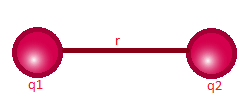$\vec{F}=\frac{1}{4\pi\epsilon _{0}}\frac{q_{1}q_{2}}{\left | \vec{r}\right |^{2}}\hat{r}$

Here k= 1/4πε0 = 9 x 109 Nm2/C2

q1 and q2 are the charges separated by a distance r

2. Electric field

$\vec{E}=\frac{1}{4\pi\epsilon _{0}}\frac{q}{{\left |\vec{r} \right |^{2}}}\hat{r}$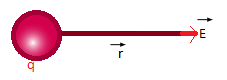3. Electric field Intensity

$\vec{E}=\vec{F}/q_{0}$

Where F is the force on q due to E

4. Electrostatic Energy

${U}=-\frac{1}{4\pi\epsilon _{0}}\frac{q_{1}q_{2}}{r}$

5. Electric Potential

$V=\frac{1}{4\pi\epsilon _{0}}\frac{q}{r}$ $dV=-\vec{E}.\vec{r}$ $V(\vec{r})=-\int_{\infty }^{\vec{r}}\vec{E}.d{\vec{r}}$

6. Electric dipole moment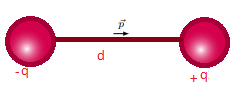$\vec{p}=q\vec{d}$

7. Potential of a dipole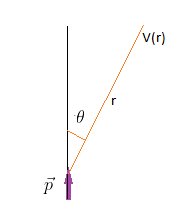$V=\frac{1}{4\pi \epsilon _{0}}\frac{pcos\theta }{r^{2}}$

8. Field of a dipole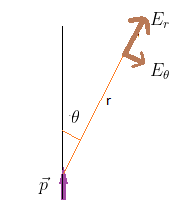$E_{r}=\frac{1}{4\pi \epsilon _{0}}\frac{2pcos\theta }{r^{3}}$ $E_{\theta }=\frac{1}{4\pi \epsilon _{0}}\frac{pcos\theta }{r^{3}}$

9. Torque on a dipole placed in the electric field

$\vec{\tau }=\vec{p}\times \vec{E}$

10. Potential energy of a dipole

$U=-\vec{p}.\vec{E}$